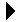World!Of
NumbersWON plate
93 |

[ March 2, 2001 ]
Palindromic Patterns using Odd & Even Tiles
Dr. Wendy Klassen (email)

Wendy Klassen went to a workshop years ago where the presenter shared an activity

You used two different colored cubes (tiles) - one for odd and one for even.
You placed the tiles next to each other somehow such that the color tile
placed was determined by adding odd or even numbers. The resulting
staircase was made up of tiles making patterns for each row, and
palindromes for each column.

Wendy has worked this with a Grade 6 class and they've come up
with all kinds of patterns and palindromes
Use two colored tiles (one for odd and one for even)
and build a tower using this information:
Odd Number (O) & Even Number (E)

 O + zero = O O + O = E O + E = O E + zero = E E + E = E E + O = O 1. Place an odd tile down. 2. To find out what tile to place underneath, you look up (odd) and to the left (zero) i.e. O + zero = O 3. Then the next tile (2nd row, 2nd tile), you look up (zero) and to the left (odd) i.e. zero + O = O 4. The first tile in the third row is O + zero = O The second tile in the third row is O + O = E The third tile in the third row is zero + O = O and so on...

The following diagram shows the first 8 rows.

O
OO
OEO
OOOO
OEEEO
OOEEOO
OEOEOEO
OOOOOOOO

It turns out that each row, column and diagonal is a palindrome!
And then there's all the patterns! Each column has a pattern of a
different number of alternating odds and evens.
Never sayNEVER ODD OR EVENagain !

One question remains. Is the palindrome property
recurrent ? Will it reappear when the staircase is
extended beyond these first 8 rows ? How many rows
must be added to find back our palindromic pattern ?A000093Prime Curios!Prime PuzzleWikipedia 93Le Nombre 93Numberland 93```

```

[TOP OF PAGE]

Patrick De Geest - Belgium- Short Bio - Some Pictures